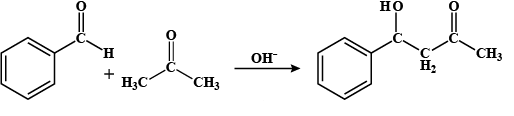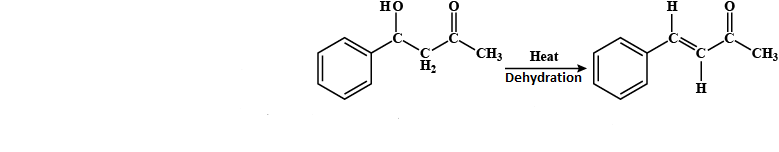QuestionAnswers

# In the following reaction, ${{\text{C}}_{6}}{{\text{H}}_{5}}\text{CHO + C}{{\text{H}}_{3}}\text{COC}{{\text{H}}_{3}}\,\xrightarrow[\Delta ]{\text{O}{{\text{H}}^{-}}}\text{ P}$ which product is formed?A. ${{\text{C}}_{6}}{{\text{H}}_{5}}\text{COH}$B. ${{\text{C}}_{6}}{{\text{H}}_{5}}\text{-CO-}{{\text{C}}_{6}}{{\text{H}}_{5}}$C. ${{\text{C}}_{6}}{{\text{H}}_{5}}\text{CH=CHCOC}{{\text{H}}_{3}}$D. ${{\text{C}}_{6}}{{\text{H}}_{5}}-\text{CO - CO - }{{\text{C}}_{6}}{{\text{H}}_{5}}$

Hint: The reaction is an example of an aldol condensation. Aldol condensation is a reaction in which the carbonyl group reacts with the enol group yielding beta-hydroxy aldehyde/ ketone. This is a type of condensation reaction.

-In the given reaction, the reactants are aldehyde and ketone and we have to find the product P formed when they both will react.
-They both will undergo aldol condensation reaction in which the following steps are followed:
Step I: firstly, the hydroxyl ion will deprotonate the aldehyde group by breaking the bond between carbon and hydrogen and releases enol ion and water i.e.Benzaldehyde Acetone 4 - hydroxy -4-phenyl but-2-one

Step II: Now, after deprotonation, the ketone attaches to the aldehyde group and forms 4 - hydroxy -4-phenyl but-2-one
Step III: Now, it will be heated and undergoes a dehydration process in which the water is released, by removal of a hydroxyl group from benzaldehyde and hydrogen from ketone that is attached to the benzaldehyde.4 - hydroxy -4-phenyl but-2-one 4-phenyl but-3-ene-2-one
-So, the product P formed is ${{\text{C}}_{6}}{{\text{H}}_{5}}\text{CH=CHCOC}{{\text{H}}_{3}}$.
So, the correct answer is “Option C”.

Note: For an aldol condensation to take place the compound should have an alpha-hydrogen i.e. hydrogen which is attached directly to the functional group. Crossed aldol condensation is the reaction in which the two different aldehyde or ketone compounds react with each other.
.